• Shuffle
Toggle On
Toggle Off
• Alphabetize
Toggle On
Toggle Off
• Front First
Toggle On
Toggle Off
• Both Sides
Toggle On
Toggle Off
Toggle On
Toggle Off
Front

### How to study your flashcards.

Right/Left arrow keys: Navigate between flashcards.right arrow keyleft arrow key

Up/Down arrow keys: Flip the card between the front and back.down keyup key

H key: Show hint (3rd side).h key

A key: Read text to speech.a keyPlay buttonPlay buttonProgress

1/35

Click to flip

### 35 Cards in this Set

• Front
• Back
 Formula for % increase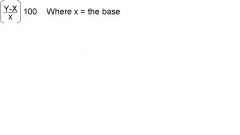* Formula for % decrease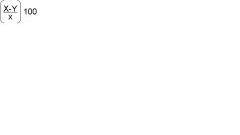Where X=the base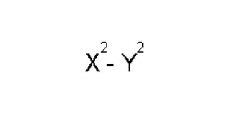What does this equal?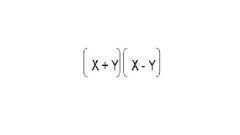answer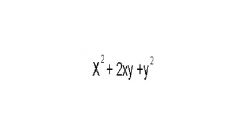What does this equal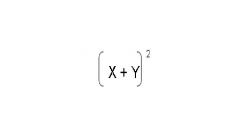answer x^2-2xy+y^2= (x-y)^2 sales price = (original price) - (%discount)(original price) total price (#of items) cost Distance Rate x time 30:60:90 triangle 1:2:square rt of 3 45:45:90 triangle leg ratios 1:sr of 2 Pi = approx 3 nCk n! / k!(n-k)! Av Speed = TD / TT P(AorB) P(A)+P(B)-P(A&B) W/ probab how do you handle exclusions? Divide factorial of # of events y the product of the exclusions 10% of 30% .10x.30 Volume of Rectangle Solid LxWxH Sum of terms = Average x # of terms Slope = Rise / run y2-y1 / x2-x1 neg slope = \ pos slope / STD = Avg of array (find mean) diff btwn each & mean Square differences Find mean of squared differences Probability formula # favorable outcomes / # possible outcomes 6C2 15 4C2 4 16C4 = 16C12 = multiple of 3 sum of digits is multiple of 3 multiple of 4 last two digits is a multiple of 4 multiple of 6 sum of digits is a multiple of 3 and last digit even multiple of 9 sum of digits is a multiple of 3 and sum of last two digits are multiple of 4 ie 135 % formula = part = percent x whole Percent increase = amount of increase / original whole then x 100 Percent decrease = amount of decrease / original whole then x 100 What is the average formula avg = sum / # of terms sum = avg x # of terms Avg of evenly spaced #s avg of min + max How do you count consecutive numbers? Max - Min +1 Ratio formula ratio = of / to